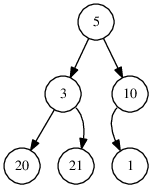Your browser (Unknown 0) is no longer supported. Some parts of the website may not work correctly. Please update your browser.

#### TreeHeight

Compute the height of a binary tree.

In this problem we consider binary trees, represented by pointer data structures.

A binary tree is either an empty tree or a node (called the root) consisting of a single integer value and two further binary trees, called the left subtree and the right subtree.

For example, the figure below shows a binary tree consisting of six nodes. Its root contains the value 5, and the roots of its left and right subtrees have the values 3 and 10, respectively. The right subtree of the node containing the value 10, as well as the left and right subtrees of the nodes containing the values 20, 21 and 1, are empty trees.A path in a binary tree is a non-empty sequence of nodes that one can traverse by following the pointers. The length of a path is the number of pointers it traverses. More formally, a path of length K is a sequence of nodes P, P, ..., P[K], such that node P[I + 1] is the root of the left or right subtree of P[I], for 0 ≤ I < K. For example, the sequence of nodes with values 5, 3, 21 is a path of length 2 in the tree from the above figure. The sequence of nodes with values 10, 1 is a path of length 1. The sequence of nodes with values 21, 3, 20 is not a valid path.

The height of a binary tree is defined as the length of the longest possible path in the tree. In particular, a tree consisting of only one node has height 0 and, conventionally, an empty tree has height −1. For example, the tree shown in the above figure is of height 2.

## Problem

Write a function:

class Solution { public int solution(Tree T); }

that, given a non-empty binary tree T consisting of N nodes, returns its height. For example, given tree T shown in the figure above, the function should return 2, as explained above. Note that the values contained in the nodes are not relevant in this task.

## Technical details

A binary tree can be given using a pointer data structure. Assume that the following declarations are given:

class Tree { public int x; public Tree l; public Tree r; }

An empty tree is represented by an empty pointer (denoted by null). A non-empty tree is represented by a pointer to an object representing its root. The attribute x holds the integer contained in the root, whereas attributes l and r hold the left and right subtrees of the binary tree, respectively.

For the purpose of entering your own test cases, you can denote a tree recursively in the following way. An empty binary tree is denoted by None. A non-empty tree is denoted as (X, L, R), where X is the value contained in the root and L and R denote the left and right subtrees, respectively. The tree from the above figure can be denoted as:

(5, (3, (20, None, None), (21, None, None)), (10, (1, None, None), None))

## Assumptions

Write an efficient algorithm for the following assumptions:

• N is an integer within the range [1..1,000];
• the height of tree T (number of edges on the longest path from root to leaf) is within the range [0..500].